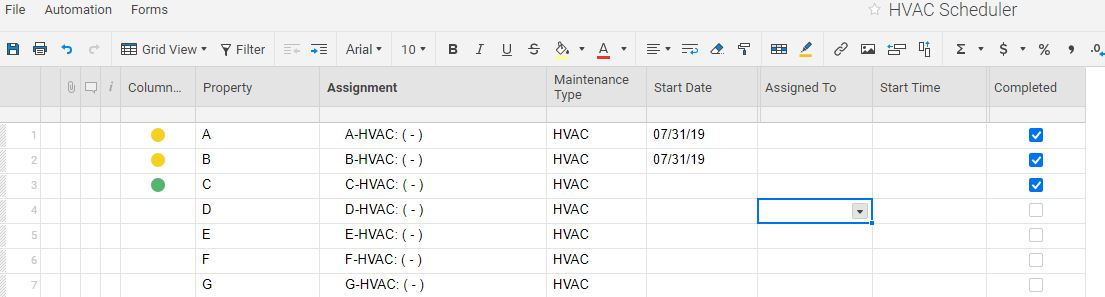# Formula for RYG symbols with dates and checkbox

I'm trying to combine two formulas to automate RYG symbols.  The first, this one, works fine to automate the symbols to be either red or yellow.  Row #1 in the screenshot.  The purpose is to keep the symbol red if the date column is either blank or empty, and yellow if there is a date in the column.

=IF(ISBLANK([Start Date]@row), "Red", IF([Start Date]@row < TODAY(), "Red", IF([Start Date]@row = TODAY(), "Yellow", IF([Start Date]@row > TODAY(), "Yellow"))))

What I want to be able to do is have the symbol turn green if a checkbox column is checked.  This formula works by itself to do this. Row #3 in the screenshot.

=IF(Completed@row = 1, "Green")

I tried to combine the formulas into one so that if the "Completed" box is checked, the symbol will change to green and override the rest of the formula.  This is how I tried to combine them, but it won't turn the symbol Green. Row #2 of the screenshot.

=IF(ISBLANK([Start Date]@row), "Red", IF([Start Date]@row = TODAY(), "Yellow", IF([Start Date]@row > TODAY(), "Yellow", IF(Completed@row = 1, "Green"))))

Any suggestions for combining these so that when the "Completed" column is checked, the symbol will turn to green?Tags: